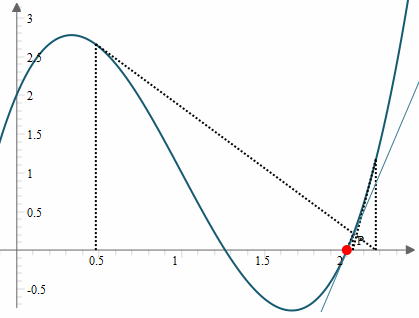Search IntMath
Close

# Newton’s Method interactive graph

By Murray Bourne, 30 Jul 2015

I added a new interactive graph that helps explain how Newton's Method works. You can find it here:

Newton's Method uses differentiation to solve non-linear equations and is especially useful when we can't factor the equation. It's very commonly used by computers when solving math-based problems.

The graph allows you to explore 4 different functions, and to see what is going on when applying Newton's Method to those functions.

You can see one of the big traps for the unwary. It doesn't always work out that the root you get is the closest one.

For example, the initial guess in this case was x0 = 0.5. The closest root is near x = 1.3, but the actual root found is one near x = 2.Also, strange things can happen if our first guess is too close to a local maximum or minimum. Try it!

## Visuals are important

I remember when I first learned Newton's Method. We happily differentiated and substituted. The examples were always "nice" with straightforward solutions. I wasn't really sure what was going on, even though I could apply the algorithm.

A visual representation is very useful for a better understanding of this concept.

### Comment Preview

HTML: You can use simple tags like <b>, <a href="...">, etc.

To enter math, you can can either:

1. Use simple calculator-like input in the following format (surround your math in backticks, or qq on tablet or phone):
a^2 = sqrt(b^2 + c^2)
(See more on ASCIIMath syntax); or
2. Use simple LaTeX in the following format. Surround your math with $$ and $$.
$$\int g dx = \sqrt{\frac{a}{b}}$$
(This is standard simple LaTeX.)

NOTE: You can mix both types of math entry in your comment.